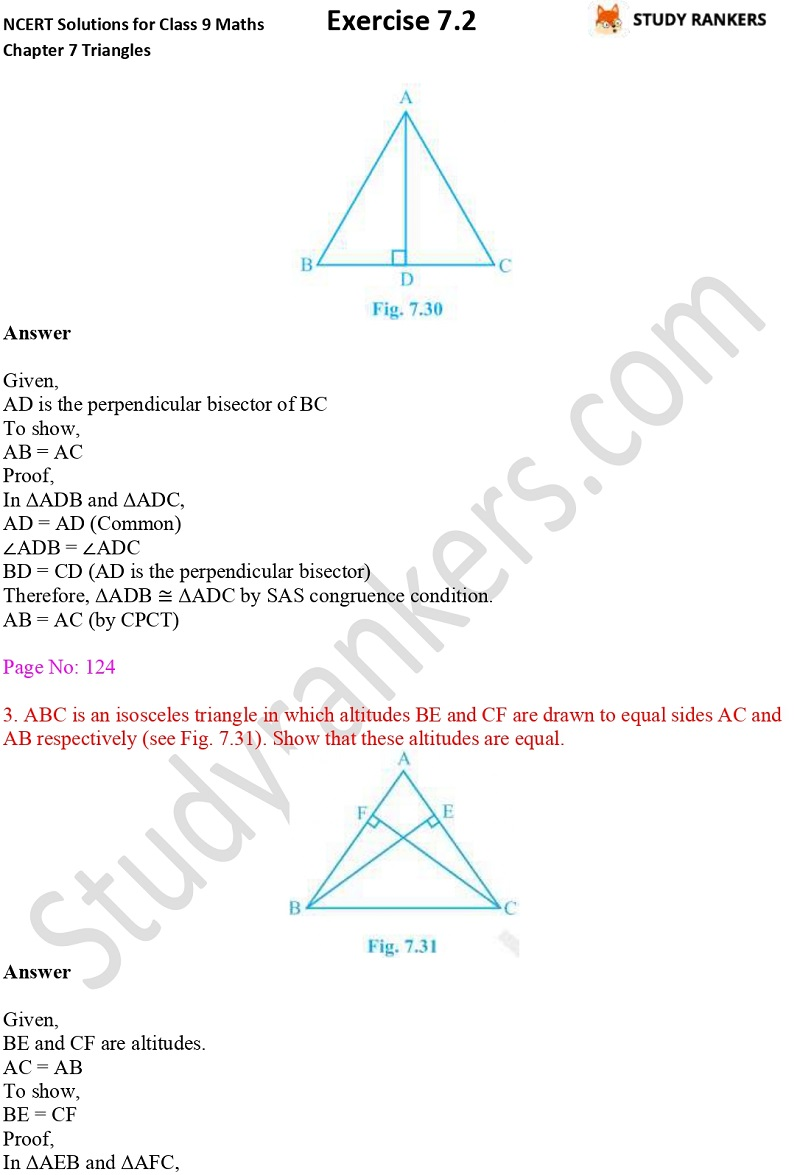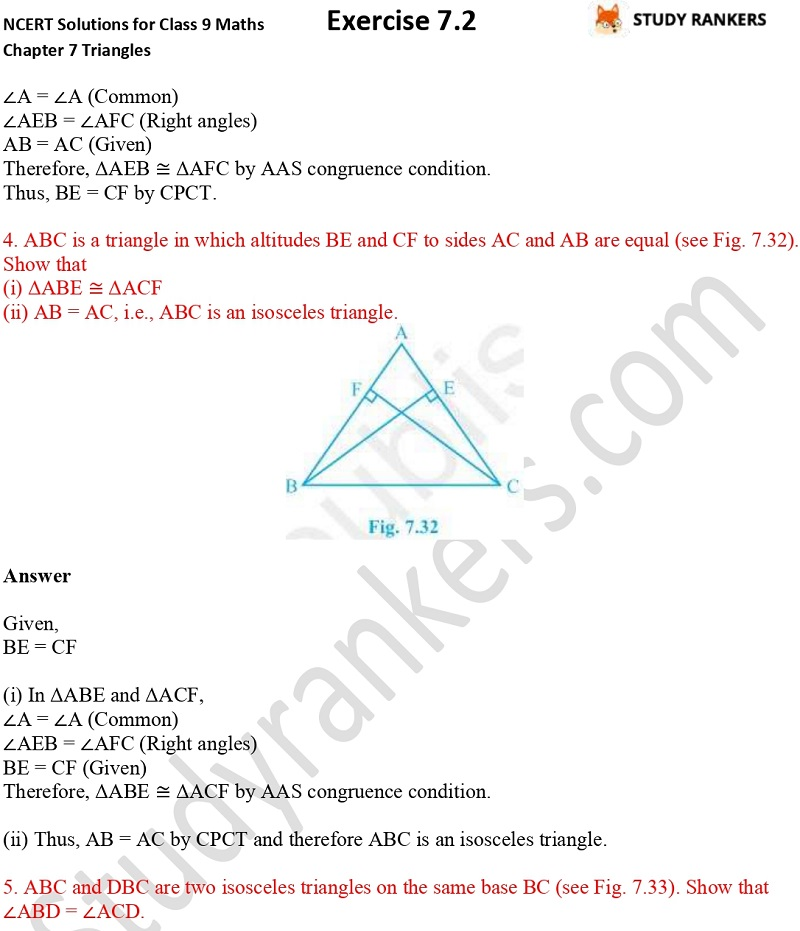>

## NCERT Solutions for Class 9 Maths Chapter 7 Triangles Exercise 7.2

You can find NCERT Solutions of Chapter 7 Triangles 7.2 here that will help you in finding the solutions of difficult questions. Class 9 Maths NCERT Solutions provided here are step by step so a student can understand them easily. These NCERT Solutions are updated as per the latest syllabus of CBSE that will be useful for improving your problem solving skills.

Exercise 7.2 has total eight questions which are about showing a triangle isosceles, right angled etc.X